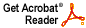# Noncoincidence of the strict and strong operator topologies

Joel H. Shapiro

Proc. Amer. Math. Soc. 35 (1972), 81--87

Abstract: Let E be an infinite dimensional subspace of C(S), the space of bounded continuous functions on a localy compact Hausdorff space S. For a regular Borel measure m on S, each element of E can be regarded as a bounded linear operator on L^p(mu) for 1<=p<infinity. The main result of this paper states that the strong operator topology thus induced on E is properly weaker than the strict topology. For E the space of bounded analytic functions on a plane region, and m=Lebesgue area measure on this region, this answers a question raised by Rubel and Shields in: The space of bounded analytic functions on a plane region (Ann. Inst. Fourier Grenoble 16 (1966), 235--277). Our methods provide information about the p-summing properties of the of the strict topology on subspaces of C(S) and the bounded weak-star topology on conjugate Banach spaces.
Download .pdf file (1.1MB)